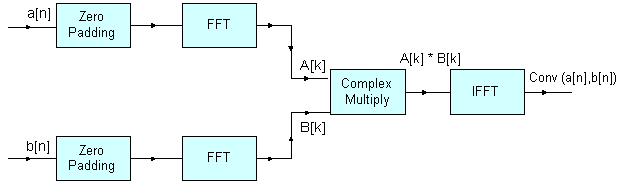CMSIS-DSP  Version 1.7.0 CMSIS DSP Software Library
Convolution Example
Description:
Demonstrates the convolution theorem with the use of the Complex FFT, Complex-by-Complex Multiplication, and Support Functions.
Algorithm:
The convolution theorem states that convolution in the time domain corresponds to multiplication in the frequency domain. Therefore, the Fourier transform of the convoution of two signals is equal to the product of their individual Fourier transforms. The Fourier transform of a signal can be evaluated efficiently using the Fast Fourier Transform (FFT).
Two input signals, `a[n]` and `b[n]`, with lengths `n1` and `n2` respectively, are zero padded so that their lengths become `N`, which is greater than or equal to `(n1+n2-1)` and is a power of 4 as FFT implementation is radix-4. The convolution of `a[n]` and `b[n]` is obtained by taking the FFT of the input signals, multiplying the Fourier transforms of the two signals, and taking the inverse FFT of the multiplied result.
This is denoted by the following equations:
``` A[k] = FFT(a[n],N)
B[k] = FFT(b[n],N)
conv(a[n], b[n]) = IFFT(A[k] * B[k], N)```
where `A[k]` and `B[k]` are the N-point FFTs of the signals `a[n]` and `b[n]` respectively. The length of the convolved signal is `(n1+n2-1)`.
Block Diagram:Variables Description:
• `testInputA_f32` points to the first input sequence
• `srcALen` length of the first input sequence
• `testInputB_f32` points to the second input sequence
• `srcBLen` length of the second input sequence
• `outLen` length of convolution output sequence, `(srcALen + srcBLen - 1)`
• `AxB` points to the output array where the product of individual FFTs of inputs is stored.
CMSIS DSP Software Library Functions Used: